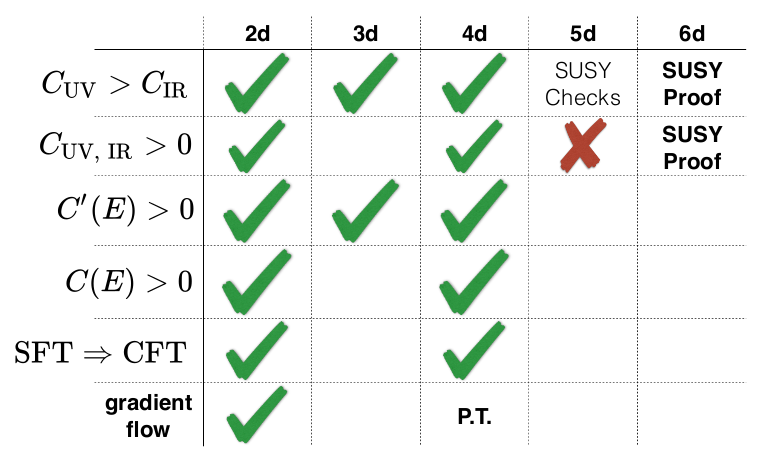Thomas Dumitrescu gave a very clear summary of the status of several conjectures and theorems concerning the renormalization group flow in various dimensions at Strings today. These are usually known as c-theorems, a-theorems or F-theorems. Here are some notes that summarize some aspects of Thomas' talk.

Let's start with basic comments about degrees of freedom (DOF) in quantum mechanics. In a finite system, it is usually easy to identify those DOF, for instance counting the number of arguments of the wave-function, or of the Hilbert space is known, by determining its dimension. When instead we consider a quantum field theory (QFT), the number of DOF is infinite. In this infinity, however, not all DOF are equally important for us: we are mostly interested in the physics at long distance. In fact, a QFT can in general be obtained as a continuum limit of a discrete system, and infinitely many microscopic DOF are in reality uninteresting, because depending on the cut-off: they are non-universal, and as such we would like to forget them. This is the central and powerful idea of renormalization group (RG) flow.

In the RG framework, the "heavy" DOF decouple from the long-distance physics as the energy cut-off is decreased, and this can be seen as a flow inside the space of theories (it is important to note that this flow is by nature irreversible). Therefore the infrared (IR) limit is interesting in this respect. On the other side of the flow, is the theory is still well-defined in the ultraviolet (UV) limit, it is called UV-complete, and it is also a compelling subject of study. Both endpoints are expected to be scale-invariant, almost by definition. However, it is important to point out at least two provisos here, that contain crucial questions about the nature of the RG flow:

• There might not exist a unique fixed endpoint. One can for instance imagine a limiting cycle in the IR. Can we construct explicit examples of this behaviour?
• Scale invariance implies in many cases that the endpoints will enjoy conformal invariance. But when exactly does this happen?

Let's come back to the RG flow as a process of forgotting degrees of freedom. To quantify this, we need some counting function (one could imagine just counting the number of fields, but for non-free theory this is not well-defined) $c(E) \geq 0$ that decreases as the energy $E$ is decreased (this is then a strong c-theorem).

### The two-dimensional case

In two spacetime dimensions, a strong c-theorem is known since 1986 when Zamolodchikov constructed a universal c-function $$c(E) \sim \langle T(x)T(0) \rangle$$ where $E^{-2} \sim x^2$ and $T$ is the energy-momentum tensor. This is always positive, and strictly decreasing away from conformal fixed points. One can even express $c$ as a height function on theory space, providing a gradient potential for the RG flow! Moreover, at conformal fixed points, $c$ coincides with the Virasoro central charge, which appears in the finite temperature free energy ($F(T) \sim c L/\beta^2$), in the Cardy formula, in the trace anomaly on curved background, in the sphere partition function, the vacuum entanglement, etc. In fact, using entanglement entropy, one can define another c-function, for which there is an elegant proof of the c-theorem (see around 18:00 in the talk above).

### Conjectures in any dimensions

Encouraged by these results in two dimensions, one might think that it is relatively easy to find c-functions in any dimension. It is in fact not the case, and many "natural candidates" are not c-functions.

If the number of dimensions is even, the trace of the energy-momentum tensor has an $a$ anomaly, which is also a coefficient in the anomalous part of the sphere partition function. Cardy conjectured in 1988 that $a_{UV} > a_{IR} \geq 0$. This conjecture was generalized in any dimension $d$ by considering an anomaly $s$ in the vacuum entanglement entropy across a sphere $S^{d-2}$. In even dimensions, $s \sim a$, while in odd dimensions $s \sim F$, where $F$ is the scheme-independant part of the partition function on $S^d$.

The status of these conjectures depend on the dimension.

• In four dimensions, after perturbative evidence in the late 80s and non-perturbatuve evidence in the late 90s from 't Hooft anomaly matching, the theorem was finally proven by Komargodski and Schwimmer in 2011 using CFT techniques.
• In three dimensions, there are also strong evidence from supersymmetric theories. A proof of the F-theorem is known using entanglement entropy techniques.
• In five dimensions, only supersymmetric CFTs are known (their existence is "guaranteed" by string theory, this also applies to six dimensions). The inequality $F_{UV}> F_{IR}$ hqs been checked in some cases, but there is no general proof, and it is not even known if $F \geq 0$ qt SCFT fixed points.
• In six dimensions, almost nothing is known about the a-theorem, despite very recent progresses in some supersymmetric theories.

A nice summary is provided at the end of the talk: